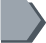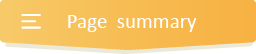Your browser does not support JavaScript!

#Division Word Problems### Fast reasonable strategies and solutions to quick solving real life division problems.

Engage your kids in this great resource with fast reasonable strategies and solutions to quick solving real life division problems grade 5.

In fact, these strategies are very useful for smart solving division problems of any kind. They include;

divisibility rules, division facts up to 12, dividing multi digit numbers by 1 digit, dividing whole numbers ending in zeroes, dividing 2 digit numbers and 3 digit numbers by 2 digit number, dividing 4 digit numbers by 2 digit numbers word problems grade 5, dividing decimals; fractions, etc.

•